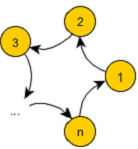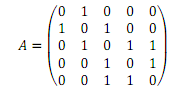### Find the number of strongly connected components

Assignment Help Other Engineering
##### Reference no: EM13669445

1. Given the following directed graph,a. Find the number of strongly connected components in the graph,

b. Compute the in-degree centralization of the graph.

c. Find an edge in the graph that removal will results in the largest number of strongly connected components in the (residual/remaining) graph. Identify nodes in each strongly connected component after removing that edge.2. Consider a circular graph which consists of n vertices 1, 2., 3,...,n. There are directed edges from vertex i to i + 1 for i = 1, 2,..,n-1 as well as a directed edge from vertex n to vertex 1. Compute the (unnormalized) closeness centrality and the (unnormalized) betweenness centrality of each node in the graph.

3. Consider the random graph G(n, p), i.e. an Erdos-Renyi graph with n vertices and each edge exists with a probability p. Prove that the expected number of isolated nodes (nodes without any incident edges) is at most n(1 - p)n-1.

4. Consider a graph G = (V, E) with the following adjacency matrix.a. Identify an edge (u, v) with the highest edge betweenness centrality and compute its edge betweenness centrality.

b. If we remove the edge (u, v), identified in the 4b, what are the connected components in the graph (list the nodes in each component).

c. Compute the modularity matrix B in which Bij = Aij - kikj/2m.

d. Consider the community structure in which each community is a connected component in 4b. Compute the modularity score associated with that community structure in G.

5. Consider an undirected graph G = (V, E) in which each edge (u, v) ∈ E exists with a probability 0 < puv ≤ 1. At the beginning of a diffusion process, the seed set S consists of exactly one node s ∈ V. For each node t ∈ V, denote by A(t) the probability that node t will be activated eventually with the seed set S = {s}. For example, A(s) = 1.

a. Prove that for any node w E V that have no path to s in G then A(w) = 0.

b. For a given edge (u, v) ∈ E, denote by

i. Auv(t) the probability that node t will be activated eventually in case we remove the edge (u, v) from G, and

ii. A+uv(t) the probability that node t will be activated eventually in case we merge two vertices It and v (that is to replace u and v with a new node r and connect r with all neighbors of u and v).

Prove that

A(t) = pA+uv(t)+ (1 - p)A-uv(t).

#### Plot a graph using scilab script and include it in

Prepare a Scilab script to evaluate the volumetric flux of smoke in a plume produced by a fire of given HRR Q'' The plume loses a percent of its heat by radiation and ß percen

#### What is the total development airflow

Determine the required airflow for diesel dilution at the face of the heading if the requirement is for 0.05 m3/s of air per kW of diesel power and what is the total developme

#### What is the friction factor of the tunnel

determine the resistance of the tunnel. If 70 m3 /s of air is passed along this tunnel what would be the pressure drop along the tunnel and what is the friction factor of the

#### Calculate volumetric flow rate and mass flow rate

Calculate the volumetric flow rate and the mass flow rate(ENMIN 5023 Mine Ventilation)- determine the average flow velocity of the air and the air quantity flowing -  what par

#### Problem - loan services at a bank

Will hiring the second loan expert decrease the average total time a customer spends in the bank for completing his/her loan request - Human Resources Manager suggested rep

#### Green cross-docking

how you determine the expected amount of CO2 generated per hour due to inbound operations when you start using forklift alternative 1?- Define the queueing system by describin

#### Define the queueing system

Define the queueing system by describing the customers, arrival rate, expected interarrival time, server(s), service rate, and expected service time. Use Kendall's notation

#### Write a position piece on the future of energy storage

Write a position piece on the future of energy storage. The length of the article should be maximum 10 sentences and should concentrate on predicting which energy storage te

### Write a Review